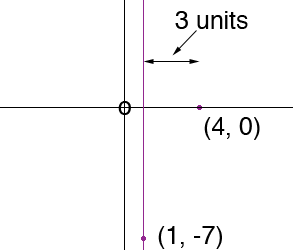Quandaries and Queries Name: Lindsey Who is asking: Student Level of the question: Secondary Question: I need to find the quadratic equation in the form: f(x)=a(x2 - (-b/a)x + (c/a)) The vertex is (1, -7), the roots are (4, ?) I need to find the other root but I don't know where to begin. My answer key says the other root is (-2). How is this possible? Hi Lindsey, I expended the equation to put it back into the generic form f(x) = a x2 + b x + c I can show you two ways to solve this problem. One solution is geometric and is the method I prefer so I am going to do it first. This quadratic equation is the equation of a parabola that either opens upward if a > 0 or downwards if a < 0. In either case it is symmetric about the vertical line through the vertex. In your example this is the line x = 1. To the right of x = 1, the graph crosses the x-axis at x = 4.Thus the graph crosses the x-axis 3 units to the right of the line of symmetry. By the symmetry the graph must cross the x-axis 3 units to the left of the line of symmetry, that is at x =  -2. The second method of solution is algebraic. To use this method you need to know that the vertex of the parabola f(x) = a x2 + b x + c occurs at x = -b/2a Since the vertex is at (1, -7) this gives 1 = -b/2a or b = -2 a (equation 1) The y-coordinate of the vertex is -7 so a 1 2 + b 1 + c = -7 or a + b + c = -7 (equation 2) Finally there is a root at x = 4 so 42a + 4b + c = 0 that is 16 a + 4 b + c = 0 (equation 3) Solve equations 1, 2 and 3 for a, b and c. Penny